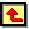# Chapter 3 : REMPI studies of H atoms and CH3 radical species in a HF-CVD reactor

This chapter contains the results of REMPI studies of H atom and CH3 radical species in a hot filament CVD reactor, with the addition of CH4 or C2H2 to a H2 gas flow.  Experimental considerations were discussed in chapter 2.

The gas-phase chemistry of H2 and CH4 input mixtures have been previously studied in some detail with H atoms and CH3 radicals being widely recognised as fundamentally important species in the hot filament diamond CVD environment.

H atom (ground-state) detection in a diamond CVD environment has been carried out previously using direct absorption on the n = 2 ¬ n = 1 Lyman-a transition, LIF monitoring of the n = 3 ® n = 2 Balmer-a emission following n = 3 ¬ n = 1 two photon excitation, 3+1 REMPI and in-situ third harmonic generation (THG).  The experimental studies described in this chapter concentrate on 2+1 REMPI of both H atoms and CH3 radicals, the use and validity of which has been previous outlined in chapter 2.

3.1             H atom detection

For simplicity, the initial studies described here were carried out in a pure H2 gas phase environment rather than the dilute hydrocarbon / hydrogen gas mixture used in diamond deposition.

2+1 REMPI was used to detect H atoms, via the collection of H+ ions.  As discussed in chapter 2, the Doppler broadened H atom lineshapes may be used to assess the relative H atom number density and the local gas temperature.  Spatially resolved measurements of the H atom distribution and local gas temperature were made as a function of distance from the hot filament surface, in a chamber containing 100% H2 at 20 Torr.  Figure 3.1a shows the trend in local gas temperature (Tgas), acquired from the Doppler broadening of the H atom lineshapes, as a function of distance from the filament surface, d, with a constant filament temperature of 2375 K.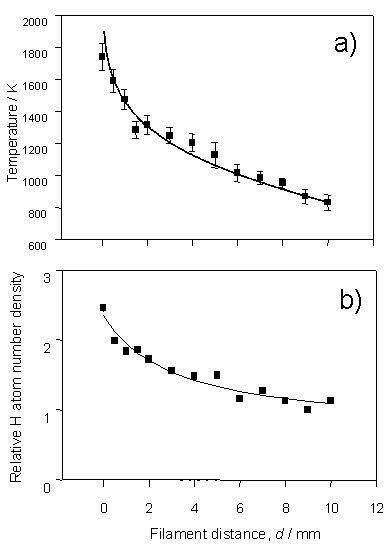Figure 3.1  H atom lineshapes yield a) local temperature dependence and b) relative H atom number density, both shown as a function of distance from the filament surface.  The data were recorded by monitoring the H atom 2s ¬ 1s two-photon transition using a chamber containing 20 Torr H2 at a flow rate of 100 sccm and a filament temperature, Tfil, of 2375 K.

The solid curve in figure 3.1a is obtained using equation 3.1, which has been used to describe the radial temperature distribution in computational models.

Td = Td=0{1-[1-(TL/Td=0)2]ln((d + Rf)/Rf)/ln(L/Rf)}0.5  Equation 3.1

Td=0 and TL in this equation are the gas temperatures in the immediate vicinity of, and at a distance L from, the hot filament surface.  The filament radius, Rf, is 125 mm (the diameter of the tantalum filament).  The correlation between the calculated temperature profile and that obtained experimentally serves to validate the local gas temperature determinations from the H atom Doppler profiles.

Figure 3.1b shows the trend in relative H atom number density, also written as [H], as a function of distance from the filament surface.  Similar radial [H] profiles have been derived using Vacuum Ultraviolet (VUV) absorption, LIF3 and THG probing, in hot filament systems.  The peak in [H] at the filament surface reinforces the claim that atomic hydrogen is formed on the surface of the hot filament, by thermal dissociation of H2, and diffuses into the bulk gas.  The recombination rate of H atoms at typical chamber pressures and temperatures is sufficiently slow that [H] in the bulk gas is greatly in excess of that predicted on the basis of equilibrium thermodynamics.

It has previously been shown that no discernable change in [H] or Tgas is detected when operating on an identical set-up with a total H2 flow rate of

50 sccm.  This supports the assertion that diffusion is the principle transport mechanism in a hot filament reactor.

Once the use of REMPI has been justified in the study of relative H atom number densities, the [H] may be monitored as a function of process conditions.  By using REMPI, [H] was found to be essentially independent of the H2 pressure.  This supports previous LIF studies and measurements of the pressure dependence as a function of filament power consumption, concluding that, at typical gas pressures and temperatures, H atoms are produced as a result of H2 bond fission on the surface of the filament.  The production of atomic hydrogen on the filament surface represents a mechanism whereby the kinetics are zero-order, with respect to the H2 pressure.  With the increase to higher H2 pressures a gradual fall in [H] is observed and attributed to the three-body homogenous recombination reaction,

H  +  H  +  M(H2)  ®  H2  +  M(H2)                            Reaction 3.1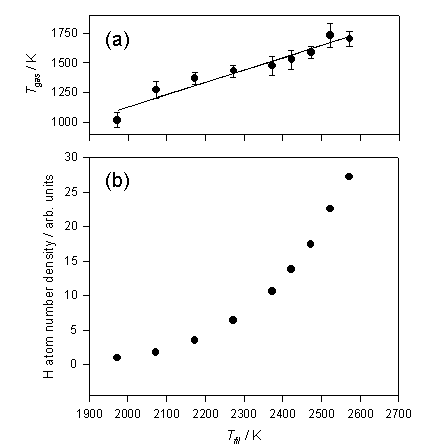Figure 3.2 shows the trends in Tgas and [H] as a function of filament temperature.

Figure 3.2  a) Tgas and b) relative H atom number densities deduced from analysis of the H atom Doppler lineshapes measured at d = 1 mm, measured as a function of filament temperature (Tfil).

Tgas scales relatively linear with Tfil, however, even at 1 mm from the filament surface the gas is ~700 K cooler than the filament surface.  Early studies by Langmuir also documented similar large temperature drops near the filament surface.  Relative H atom number densities appear to increase exponentially within the filament temperature range measured.   Plotting ln[H] against 1/Tfil (a Van’t Hoff type plot) yields a straight line, the gradient of which may be interpreted in terms of -DHDISS/R, where DHDISS has the value

240 ± 20 kJ mol-1 and is the deduced enthalpy for forming a mole of H atoms on the surface of the filament.  The surface mediated H atom formation scheme is outlined in the reversible reactions 3.2 and 3.3.

H2  +  S*  ¾  H  +  SH                                    Reaction 3.2

SH  ¾  H  +  S*                                              Reaction 3.3

These reactions describe the dissociative adsorption of H2 on an active filament surface site (S*) and H atom recombination on a hydrogen-terminated filament surface (Reaction 3.2 forward and reverse).  The reversible reaction 3.3 describes the desorption and absorption of atomic hydrogen onto the filament surface.  Changes in Tfil will influence the H atom filament production rate by affecting the mean lifetime of chemisorbed species on the filament surface, together with its energetic influence on the H atom formation reaction scheme.  The temperature of the filament also affects the fraction of available S* and the local gas phase H atom number density which, in turn, influences the fraction of active sites via the reverse of reaction 3.3.  Overall the filament acts as a means of providing active sites and a medium by which H2 molecules may attain Tfil.  In terms of a reaction scheme, the overall total reaction facilitated by the hot filament surface (i.e. the sum of reactions 3.2 and 3.3), is simply,

H2  ¾  2H.                                           Reaction 3.4

The addition of a dilute hydrocarbon to the pure H2 environment complicates matters, since, as described earlier in section 2.4, the filament surface becomes carburised and thus changes the number and type of available sites at the filament surface.

Previous H atom REMPI studies have shown that the addition of small quantities of methane causes a reduction of [H] for a given Tfil.   This may be considered consistent with the presumed change in the filament surface and a reduction in the fraction of active sites.  The loss of H atoms in the gas-phase may also be explained by analysis of the gas-phase reactions that are occurring.  Abstraction reactions, similar to that shown in reaction 3.5, provide a gas phase loss mechanism for H atoms together with a route by which methyl radicals are produced.

H  +  CH4  ¾  H2  +  CH3                               Reaction 3.5

3.2             Methyl Radical detection

The detection of methyl radicals in a diamond-depositing reactor via 2+1 REMPI has been previously explored, for the purposes of these studies the origin band of the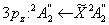transition was investigated.  Figure 3.3 shows a power normalised spectrum of theorigin band transition of CH3, recorded using a 1% CH4 / H2 gas mixture with Tfil = 2475 K, probing at d = 4 mm.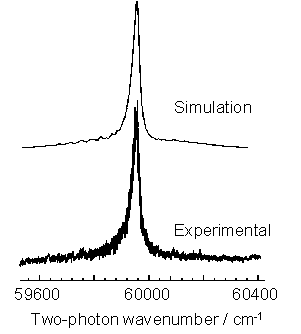Figure 3.3  2+1 REMPI spectrum of the CH3 (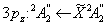) transition (lower trace) with a best-fit simulation of the origin band contour (upper trace).

The Q branch dominates the spectrum with weak underlying contributions from O, P, R and S branch structures producing an underlying pedestal.

The simulation shown in figure 3.3 (upper trace) was produced with prior knowledge of the ground and excited state spectroscopic parameters (listed in table 3.1), the nature of the excited state predissociation and the two-photon rotational linestrengths.

The rotational constants, detailed in table 3.1, define the rotational transitions for the oblate symmetric rotor CH3 radical.  For such a structure, the ground and excited state respective rotational values are given by,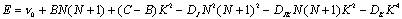Equation 3.2

The full width half maximum of a line to a given excited state N, is given by,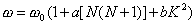,                      Equation 3.3

where w is a linestrength factor which determines the relative weights of the zero rank (scalar) and second rank tensor components of the two photon transition probability.

The only variable parameter is the rotational temperature which, in the case shown, is identified as 1150 K.  This is in agreement with the local gas temperature gained via H atom Doppler analysis for equivalent conditions and serves to highlight that local thermodynamic equilibrium (LTE) exists in this type of CVD reactor.  With an estimated collision frequency of ~108 s-1, for typical gas pressures and temperatures reached in HF-CVD reactors, the rotational temperature exhibited by CH3 radicals is equal to the gas temperature denoted by H atom Doppler lineshape analysis, and thus LTE exists.

Constant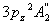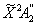Units

n0

59955(5)

cm-1

B0

9.51(7)

9.57789

cm-1

C0

4.62(3)

4.74202

cm-1

DJ

7.7

7.699

10-4 cm-1

DJK

-13.6

-13.58

10-4 cm-1

DK

6.3

6.34

10-4 cm-1

v0

12(1)

cm-1

## W

2.5(1)

a

0.05(2)

b

-1

Table 3.1  Molecular constants of the CH3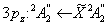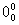band used in the simulations.  All constants taken from J. Heinze, N. Heberie and K. Kohse-Hoinghaus, Chem. Phys. Lett., 1994, 223, 305. except W, v0, and n0 which were derived from simulations of the measured spectrum.

Given that 2+1 REMPI is a viable means of monitoring relative CH3 number densities [CH3], studies were conducted to ascertain [CH3] as a function of process conditions and distance from the filament, d.  Rather than recording the entire band contour for each set of conditions, the REMPI signal was collected at a single probe wavelength within the transition.  In order to convert the CH3 REMPI signal into [CH3], the local gas temperature needs to be known as a function of d together with the application of a correction factor that recognises that both, the population of the probed n = 0 level and the origin band contour are temperature dependent.  The local gas temperature profile, as a function of d, is known from H atom Doppler lineshape analysis and is shown in figure 3.1a for a given Tfil.  The two temperature dependant correction factors, by which the measured CH3 REMPI signal must be divided in order to attain [CH3], are summarised in figure 3.4.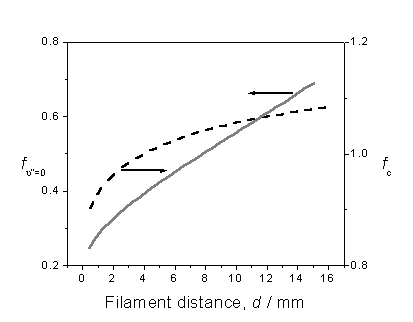Figure 3.4  Plot illustrating the variation in correction factors fc and fn”=0 with filament distance, assuming local gas temperatures provided by H atom Doppler lineshape analysis.

Factor fc is obtained by calculating the CH3 () origin band contour for several different rotational temperatures, within the range 600 – 1700 K, then determining the fraction of the total band contour that falls within the bandwidth of the probe laser.  The probe laser is assumed to have a Gaussian profile centred at a two-photon energy of 59950 cm-1.  The other factor, fn”=0, recognises that the REMPI probe wavelength only samples the fraction of methyl radicals that are present in the ground vibrational state.  Assuming that LTE exists, the temperature dependence of fn”=0 is calculated given knowledge of the CH3 normal mode frequencies.  The CH3 radical has two singly degenerate fundamental modes, a symmetric stretch vibration

(n1 = 3004.4 cm-1) and an out-of-plane bend (n2 = 606.5 cm-1), together with two doubly degenerate modes, an asymmetric stretch (n3 = 3160.8 cm-1) and an in-plane bend (n4 ~1400 cm-1).

The measured REMPI signals will only provide a reliable measure of the total CH3 relative number density if the population in all of the populated vibrational states is sampled with equal efficiency.  However, in the case of the CH3() transition, the out-of-plane bending vibration (n2) participates in the majority of the thermally populated levels with n” > 0.  This, together with the Franck-Condon requirement that Dn2 must be even, ensures that all of the most significant hot band transitions in the 2+1 REMPI spectrum of CH3 will be blue shifted with respect to the origin band.  Therefore, the measured CH3 REMPI signals must be corrected to reflect the trend in fn”=0.

The correction factors were applied to CH3 REMPI signals collected from studies focusing on [CH3] as a function of process conditions and filament distance.  Addition of trace quantities of CH4 or C2H2 to a 100 sccm flow of H2 was studied in terms of CH3 production.  Figure 3.5 shows the trend in [CH3] as a function of % CH4 or C2H2 addition.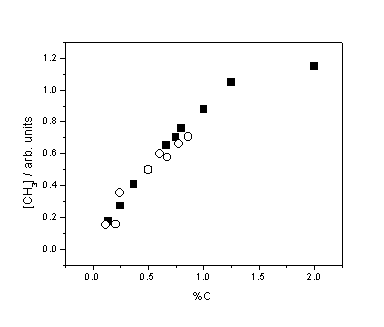Figure 3.5  Relative CH3 number density as a function of %C from CH4 (¢) and C2H2 () addition.  Measurements were obtained with Tfil = 2475 K and d = 4 mm.  The data points for the CH4 and C2H2 sets have been separately scaled vertically, in practice [CH3] is approximately 2.5 times higher for CH4 than C2H2 for the same %C input.

The linear trend in [CH3] at low CH4 addition (<2%) is in accord with several previous measurements and is clearly different from the previously identified constant [H] as a function of added H2.  This reinforces the claim that methyl radicals, unlike H atoms, are principally formed in the gas-phase and not on the hot filament surface.

Monitoring the CH3 REMPI signal, as a function of Tfil, reveals that in comparison to the H atom dependence the CH3 REMPI signal rises less steeply.  Figure 3.6 shows how both the H atom number density and CH3 REMPI signal depend on Tfil.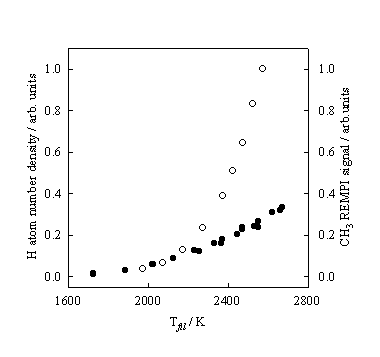Figure 3.6  H atom number densities (¡) and CH3 REMPI signal intensities (l) measured at, respectively, d = 4 and 2 mm, and 20 Torr pressure, plotted as a function of Tfil.

The design of the Hot-filament reactor and REMPI both allow spatially resolved number density measurements to be undertaken.  In this case the filament was translated vertically, with respect to the laser focal position and REMPI probe, to determine relative CH3 number densities as a function of distance from the filament.

The collection of spatially resolved H atom number densities has previously been discussed in sub-section 2.3.  Figure 3.7 shows the radial dependence of the measured CH3 REMPI signal for a 1% CH4 in H2 gas mixture measured at a pressure of 20 Torr and Tfil = 2475 K.  The plot shows how the measured signal (l) is corrected, by inclusion of the correction factors fc and fn”=0 described above, to form a relative number density measurement (¡).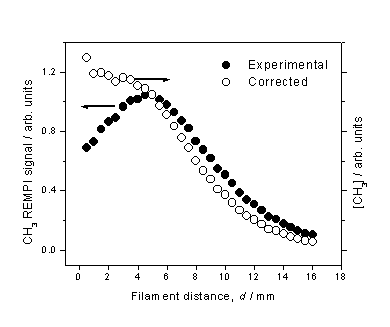Figure 3.7  CH3 REMPI signal (l) measured as a function of d from a 1% CH4 in H2 gas mixture.  The total CH3 relative number density (¡) is obtained by scaling the measured REMPI signal by the temperature dependent correction factors.

The peak in measured CH3 REMPI signal is in accord with studies by Kruger, Zare et al. using cavity ring down spectroscopy (CRDS) to monitor a column of CH3 density in a hot filament reactor operating on a CH4/H2 gas mixture.  We note, however, that this peak becomes more of a plateau after correction for the temperature dependant sampling efficiency.  Nonetheless, this observation is in marked contrast with the spatially resolved H atom number density measurements, and may be explained by realisation that the CH3 number density is largely determined by the gas-phase equilibrium,

H  +  CH4  ¾  CH3  +  H                                 Reaction 3.6

Although the H atom number density decreases monotonically with increasing d, the total gas number density (and thus CH4 number density) increases.  This is due to the inverse dependence between number density and gas temperature (for a constant pressure).

3.3       C2H2 gas-phase chemistry

Previous growth studies have demonstrated that the deposition of diamond may occur with equal ability regardless of the hydrocarbon used.  Gas-phase simulations have been used to study the species and reaction scheme.  A number of inconsistencies have been found, particularly in the comparison of CH4 and C2H2 as hydrocarbon sources1.  Growth studies have shown that growth by C2H2 occurs with similar deposition characteristics (growth rate and quality) to growth from equivalent carbon containing CH4 gas mixtures.  Close inspection of a C / H reaction scheme (such as provided in GRI-Mech) reveals that there is not a viable route for the production of CH3 radicals from C2H2 feedstock gas at the elevated temperatures typically found in the CVD environment.  This has led some to conclude that CH3 production from C2H2 must be facilitated by heterogeneous conversion on the reactor walls, thereby providing a route to C-C bond breakage9.

With the spatial resolution provided by REMPI and the reactor set-up, spatially resolved CH3 REMPI signals, and hence, calculated number densities, were obtained for a C2H2/H2 gas mixture.  Obtaining the relative CH3 number density as a function of d provides an insight into the gas-phase chemistry, and serves to substantiate or invalidate the conclusion that CH3 is formed via a gas-surface reaction at the reactor walls.  In the experimental set-up the reactor walls are remote (>6 cm from filament) and constantly cooled.  Figure 3.8 shows the measured CH3 REMPI signal obtained from a 0.5% C2H2 in H2 gas mixture as a function of d.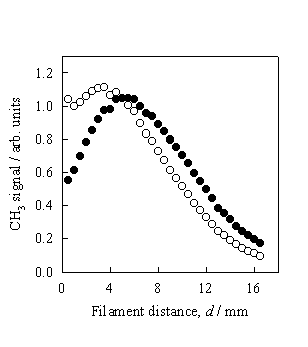Figure 3.8  CH3 REMPI signal (l) measured as a function of d from a 0.5% C2H2 in H2 gas mixture.  The total CH3 relative number density (¡) is obtained by scaling the measured REMPI signal to the temperature dependent correction factors.

The measurements were taken at Tfil = 2475 K and a chamber pressure of 20 Torr.  Clearly the trend shown in figure 3.8 is in close agreement to that obtained using a 1% CH4 in H2 gas mixture (figure 3.7) and serves to invalidate previous conclusions of CH3 production from C2H2 via heterogeneous conversion.

Experimentally determined CH3 number density variations with hydrocarbon/H2 mixing ratio, Tfil (Figure 3.9) and as a function of radial distance, all showed little sensitivity to whether the carbon is introduced as CH4 or C2H2.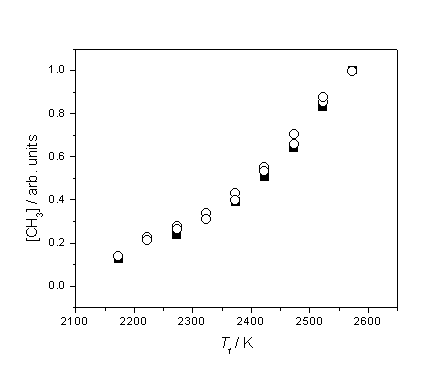Figure 3.9  Plot highlighting the similar trend of increasing [CH3] with Tfil using 1% CH4 in H2 (¢) and 0.5% C2H2 in H2 (), probing at d = 4 mm.  The two datasets have been vertically re-scaled to coincide at Tfil = 2575 K.

Clearly the two hydrocarbons must produce CH3 by different routes, but both must be explained in terms of gas-phase chemistry.  Toyoda et al.2 have argued that a sequence of H and H2 addition steps along the lines of,

C2H2  +  H  +  M  ¾  C2H3  +  M                                Reaction 3.7

C2H4  +  H  +  M  ¾  C2H5  +  M                                Reaction 3.8

may provide a gas-phase route to C-C bond cleavage.

Arguably the most effective method to analyse the gas-phase chemistry, and to substantiate experimental findings, is by comparison to a computer simulation.  Working in collaboration with Moscow State University, we were able to produce experimental data, the results of which were analysed and compared to gas-phase computer simulations in Moscow.

3.4       Gas phase simulations

Computer simulations of the gas-phase and gas-surface chemistries in a diamond depositing CVD environment have previously been used to identify and analyse functional species and reactions.  This section describes gas-phase computer simulation studies carried out by Y.A. Mankelevich and N.V. Suetin of Moscow State University, Russia.  The 3-D model devised and used was tailored to the Bristol Hot filament CVD reactor used in the REMPI studies, and was used to assist in the unravelling of the complex gas-phase chemistry.  Previous studies of the diamond depositing HF-CVD gas-phase environment have implemented 1-D and 2-D models, however these have yielded poor correlation with experimental results.  3-D modelling of the gas-phase environment was expected to describe the species and heat transfer more accurately.

The model consists of three sections that describe the activation of the gas mixture (i.e. gas heating, filament activation), the gas-phase processes (heat and mass transport and reaction kinetics) and the gas-surface growth process at the substrate.  Gas-phase chemistry and thermochemical input is provided by the GRI-Mech 3.0 reaction mechanism, which details gas-phase reaction mechanisms for C / H / O reaction mixtures.  For the present application the reaction scheme was modified by removing all oxygen containing species and reactions.  Spatial distributions of the gas temperature, the mass and heat flux, and species concentrations, are calculated by integrating the conservation equations for mass, momentum, energy and species concentrations in Cartesian co-ordinates, with appropriate initial and boundary conditions, thermal and caloric equations of state, until a steady state regime is achieved.

The Cartesian co-ordinates used by the model are set to mimic that of the Bristol reactor with the (0,0,0) point defining the centre of the filament.  The z-axis is set parallel to the gas flow direction and perpendicular to the filament, the x- and y- axes lying respectively orthogonal to the filament and gas flow, and parallel to the filament.  The model allows a degree of flexibility in the setting of certain parameters.  In the studies detailed here the less well known parameters Q, (the rate of atomic hydrogen production at the filament surface) and DT, (the temperature difference between the filament surface and the gas in the immediate vicinity of the filament) were varied.  The temperature drop may also be expressed in terms of the near-filament temperature, Tnf, where DT = Tfil - Tnf.

3.5       3-D calculations of CH4/H2 and C2H2/H2 chemistry

Calculations of the methyl radical number density, as a function of distance from a filament, were compared to the previously described spatially resolved CH3 REMPI measurements taken with dilute CH4 or C2H2 in H2 gas mixtures.

Figure 3.10 shows CH3 radical concentrations predicted by a full 3-D model calculation, as a function of distance z from the filament, for different Tnf and Q.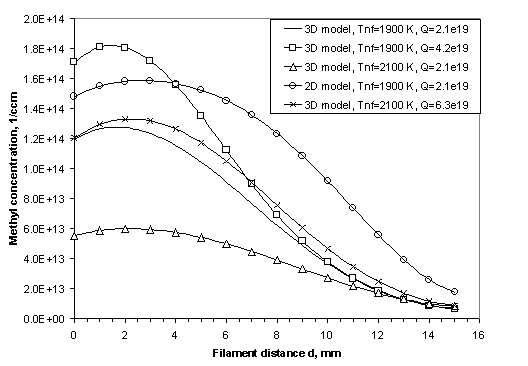Figure 3.10  Calculated CH3 radical concentration profiles in the model illustrating the effects of Q, Tnf and the discrepancies introduced by a lower dimension calculation.

This diagram is included here simply to highlight the errors that may be introduced by using a lower dimension calculation and to show the general CH3 radial concentration trend observed with changes in Q and Tnf.

Figure 3.11 compares the experimental CH3 radical relative number density measurements, obtained using both 1% CH4 in H2 and 0.5% C2H2 in H2 gas mixtures, with the best fit 3-D model calculations which assume Q = 0.6 ´ 1019 cm-2 s-1 and Tnf = 2000 K (DT = 475 K).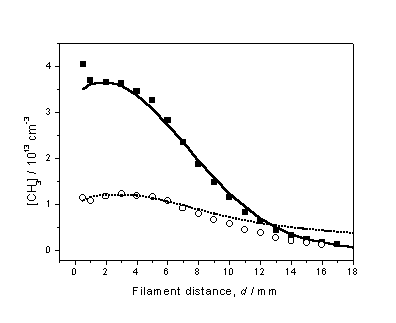Figure 3.11  Experimentally determined d dependent [CH3] profiles obtained using 1% CH4 in H2 (¢) and 0.5% C2H2 in H2 () gas mixtures, with Tfil = 2475 K.  To establish a common vertical scale for this plot, measurements were made while switching between CH4 and C2H2, while maintaining a constant Tfil.  The 3-D model calculations are represented by the solid and dashed curves and, implicitly, yield the absolute number densities shown on the left-hand axis.

With both the Q and Tnf parameters established, further investigations into the C2H2/H2 gas-phase chemistry could occur.  Inspection of the GRI-Mech 3.0

C / H reaction scheme reveals large variations in the chemistry.  This is due to dependence on the local gas temperature and therefore the region studied in the reaction chamber.  Table 3.2 outlines a number of reactions involving the production and loss of C2H2 together with calculated reaction rates for a given gas temperature.

Reaction Rate / cm-3 s-1

Reaction                                                                730 K                       1200 K                     1750 K                     2000 K

H + C2H2+ M ® C2H3 + M                         1.85E +16                4.00E +15                4.07E +14                1.64E +14
C2H3 + M
® H + C2H2 + M                         3.72E +12                1.23E +15                1.93E +16                3.23E +16
H + C2H3
® H2 + C2H2                              1.05E +16                3.34E +15                5.24E +15                6.07E +15
H2 + C2H2
® H + C2H3                              7.15E +1                  2.54E +9                  8.58E +12                6.97E +13
Total: C2H2 ® C2H3                                               8.00E +15                -5.73E +14               -2.41E +16               -3.82E +16

H + C2H4 ® H2 + C2H3                              7.73E +13                7.99E +15                5.78E +16                9.71E +16
C2H3 + H2
® H + C2H4                              7.64E +15                7.86E +15                3.35E +16                5.47E +16
H + C2H3 + M
® C2H4 + M                        3.12E +14                2.21E +13                8.58E +12                5.93E +12

C2H4 + M ® H + C2H3 + M                        0                              5.25E +6                  1.15E +12                2.38E +13

Total: C2H3 ® C2H4                                               7.87E +15                -1.10E +14               -2.42E +16               -4.25E +16

C2H4 + M ® H2 + C2H2 + M                       4.14E +1                  8.76E +10                5.94E +14                4.40E +15
H + C2H4 + M
® C2H5 + M                        5.02E +15                6.14E +14                3.55E +13                1.20E +13
C2H5 + M
® H + C2H4 + M                        3.15E +11                2.29E +14                1.47E +15                1.52E +15
H + C2H5
® H2 + C2H4                              4.32E +13                4.01E +13                3.13E +13                2.37E +13
H2 + C2H4
® H + C2H5                              0                              2.50E +7                  5.88E +10                4.25E +11
Total: C2H4
® C2H5                                               4.97E +15                3.45E +14                -1.47E +15               -1.53E +15

CH3 + CH3 + M ® C2H6 + M                      4.22E +14                2.77E +14                3.30E +13                1.13E +13
C2H6 + M
® CH3 + CH3 + M                      6.74E +2                  5.09E +11                8.01E +14                3.02E +15
CH3 + CH3
® H + C2H3                             3.73E +11                3.25E +13                1.47E +14                1.80E +14
H + C2H5
® CH3 + CH3                             5.39E +15                4.82E +15                3.13E +15                2.18E +15
Total: C2H6/C2H6
® CH3                        4.97E +15                4.51E +15                3.75E +15                5.00E +15

H + C2H6 ® H2 + C2H5                              5.94E +14                4.55E +15                4.76E +15                3.86E +15

C2H5 + H2 ® H + C2H6                              7.34E +12                8.57E +13                3.24E +14                3.97E +14
H + C2H5 + M
® C2H6 + M                        1.63E +14                1.70E +13                1.50E +12                4.98E +11
C2H6 + M
® H + C2H5 + M                        0                              2.10E +8                  1.71E +12                1.10E +13
Total: C2H6
® C2H5                                               4.23E +14                4.45E +15                4.44E +15                3.48E +15

Table 3.2  Gas-phase scheme including the major elementary steps in the C2 ® C1 conversion mechanism, together with their respective reaction rates calculated for gas temperatures observed in a hot filament reactor.  Third bodies are represented by M; due to its large number density this is typically H2.  The net reaction rates for each set of conversions are shown in bold type.

The calculated H atom number densities at the gas temperatures shown in the reaction scheme are 1.6 ´ 1014 cm-3 (730 K), 4.1 ´ 1014 cm-3 (1200 K), 5.7 ´ 1014 cm-3 (1750 K) and 6.2 ´ 1014 cm-3 (2000 K) respectively.  However, as we saw earlier (Fig. 3.1b), throughout the reaction chamber the atomic hydrogen number density is greater than that calculated purely on the basis of gas-phase thermodynamics, for any given local gas temperature.   This means that the cooler regions of the chamber away from the filament will have relatively high [H] with a low local gas temperature.  As table 3.2 shows, atomic hydrogen drives the C2 ® C1 conversion via a multi-step series of reactions at low gas temperatures (e.g. 730 K) whereas, at much higher gas temperatures closer to the filament, the reverse process dominates.  This process is summarised in figure 3.12, where the calculated d dependence of the various source terms for key species, together with Tgas is shown for a 0.5% C2H2 in H2 gas mixture.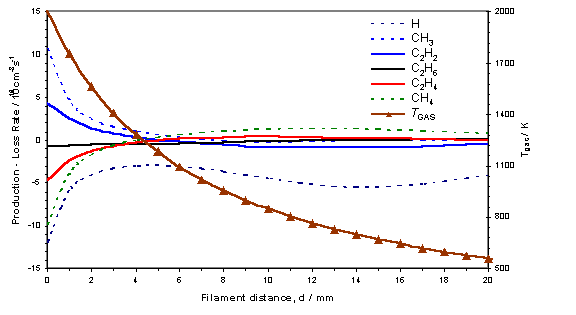Figure 3.12  3-D model calculation showing the source terms (production – loss terms in the gas-phase reaction scheme) for the key species in a hot filament reactor operating with a 0.5% C2H2 in H2 gas mixture with Tfil = 2475 K.  The local gas temperature is also shown with its corresponding scale on the right axis.

From this figure the C2 ® C1 conversion appears to maximise at d ~ 12 mm, at local gas temperatures in the range 600 – 900 K.  The process of C2 ® C1 conversion proceeds by means of the multi-step addition of hydrogen to C2H2, for example, via the scheme C2H2 ® C2H3 ® C2H4 ® C2H5 ® CH3 ® CHx (for x = 0 – 4), which occurs preferentially in cooler regions of the reactor.  The calculations show that approximately half of the reacted C2H2 is converted into CH3, with the remainder being reduced to C2H4.  The three-body recombination,

H  +  CH3  +  M  ®  CH4  +  M                                  Reaction 3.9

is sufficiently fast at low gas temperatures to allow the formation of CH4, which  may be recycled via diffusion to form CH3 close to the filament through the abstraction reaction 3.5.  This effect is also shown by C2H4, which is primarily formed in cooler regions, and may be reconverted into C2H2 close to the filament through a series of hydrogen abstraction reactions.  The produced C2H2 can then itself diffuse to cooler regions to form C2H4 and CH4.

Close to the filament surface the unimolecular decay of C2H6 makes a significant contribution to the C2 ® C1 conversion through the reaction,

C2H6  +  M  ®  CH3  +  CH3  +  M                            Reaction 3.10

In the hotter regions of the chamber, close to the filament, interconversion between various hydrocarbon species occurs readily, due to the high local gas temperature and atomic hydrogen number density.  However, this volume is relatively small in comparison to cooler regions in the reactor.  The rapid conversion of species at the filament is therefore counter-balanced by the reverse reactions in the slower but more voluminous cooler regions.  The reaction time for C2 ® C1 conversion is of the order 10-2 – 10-1 s and therefore occurs slower than the fast hydrogen shift series of reactions that operate on C1Hx and C2Hx.

The concentration distributions of key species calculated in the 3-D model reactor are shown in figure 3.13 as a function of the z-axis for 1% CH4 in H2 and 0.5% C2H2 in H2 gas mixtures.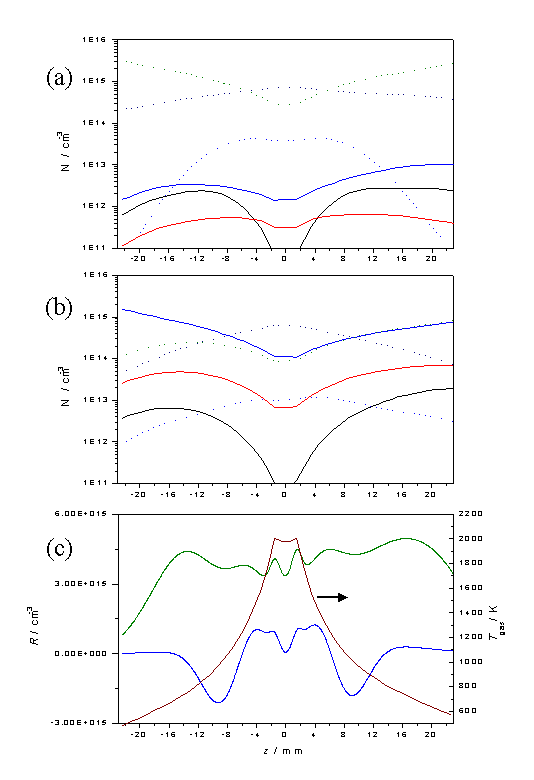Figure 3.13  Plots showing [H], [CH3], [CH4], [C2H2], [C2H4] and [C2H6] as a function of z for input gas mixtures of a) 1% CH4 in H2 and b) 0.5% C2H2 in H2 using the same legend as in figure 3.12.  Calculations were carried out assuming a chamber pressure of 20 Torr, Tnf = 2000 K and

Q = 6.0 ´ 1018 cm-2 s-1.  Plot c) shows the net C2 ® C1 conversion rate, R, for 1% CH4 in H2 (blue) and 0.5% C2H2 in H2 (green) gas mixtures, as a function of z given the temperature profile shown.

The effect of increasing the filament temperature, and thus Tnf and Q, can be seen by comparing plots equivalent to those in figure 3.13, but with

Tnf = 2300 K and Q = 1.2 ´ 1020 cm-2 s-1, these are shown in figure 3.14.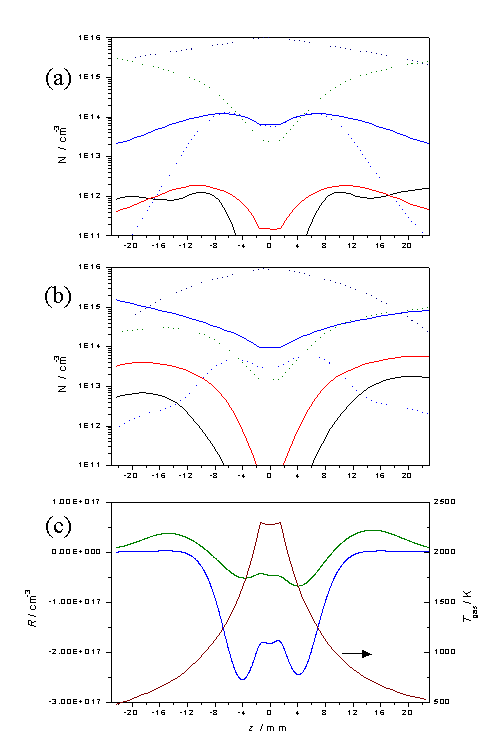Figure 3.14  Equivalent plots to those in figure 3.13, but with an increased filament temperature.

These figures serve to reinforce the view that the species number densities close to the filament, and in the region z = 6 - 10 mm, where diamond deposition would normally occur, are relatively insensitive to the source hydrocarbon.

3.6             Conclusions

This chapter has discussed the results of a 2+1 REMPI study of CH3 radicals in a hot filament CVD reactor operating on CH4 in H2 and C2H2 in H2 feedstock gases.  The results of these studies were compared to 3-D model calculations to assist in unravelling the gas-phase chemistry, in particular the C2 ® C1 conversion.  Findings suggest that C2 ® C1 conversion occurs readily via a purely gas-phase route in the cooler regions of the reaction chamber and contradicts previous assumptions that heterogeneous processes (e.g. at the reactor walls) were necessary in order to cause the conversion.

References

               D.G. Goodwin and J.E. Butler, in ‘Handbook of Industrial Diamonds and Diamond Films’,

ed. M.A. Prelas, G. Popovici and L.K. Bigelow, Marcel Dekker, New York, 1998,

pp. 527-581, and references therein.

               H. Toyoda, M.A. Childs, K.L. Menningen, L.W. Anderson and J.E. Lawler, J. Appl. Phys.,

1994, 75, 3142.

               U. Meier, K. Kohse-Hoinghaus, L. Schafer and C.P. Klages, Appl. Opt., 1990, 29, 4993.

               F.G. Celli and J.E. Butler, Appl. Phys. Lett., 1989, 54, 1031.

               F.G. Celli, H.R. Thorsheim, J.E. Butler, L.S. Plano and J.M. Pinneo, J. Appl. Phys., 1990, 68,

3814.

               Y.A. Mankelevich, A.T. Rakhimov and N.V. Suetin, Diamond Relat. Mater., 1996, 5, 888.

               M.A. Childs, K.L. Menningen, L.W. Anderson and J.E. Lawler, J. Chem. Phys., 1996, 104,

9111.

               L.L. Connell, J.W. Fleming, H.N. Chu, D.J. Vesteck, Jr., E. Jensen and J.E. Butler, J. Appl.

Phys., 1995, 78, 3622.

               D.G. Goodwin and G.G. Gavillet, J. Appl. Phys., 1990, 68, 6393.

             L. Schafer, C.P. Klages, U. Meier and K. Kohse-Hoinghaus, Appl. Phys. Lett., 1991, 58, 571.

             T. Otsuka, M. Ihara and H. Komiyama, J. Appl. Phys., 1995, 77, 893.

             I. Langmuir, J. Am. Chem. Soc., 1915, 37, 417.

             V. Zumbach, J. Schafer, J. Tobai, M. Ridder, T. Dreier, B. Ruf, F. Behrendt, O. Deutschmann

and J. Warnatz, J. Chem. Phys., 1997, 107, 5918.

             P. Zalicki, Y. Ma, R.N. Zare, E.H. Wahl, J.R. Dadomio, T.G. Owano and C.H. Kruger, Chem.

Phys. Lett., 1995, 234, 269.

             C.A. Rego, R.S. Tsang, P.W. May M.N.R. Ashfold and K.N. Rosser, J. Appl. Phys., 1990, 68,

4825, and references therein.

             P.W. May, N.M. Everitt, C.G. Trevor, M.N.R. Ashfold and K.N. Rosser, Appl. Surf. Sci.,

1993, 68, 299.

             G.P. Smith, D.M. Golden, M. Frenklach, N.W. Moriarty, B. Eiteneer, M. Goldenberg, C.T.

Bowman, R.K. Hanson, S. Song, W.C. Gardiner, Jr., V.V. Lissianski and Z. Qin,

http://www.me.berkeley.edu/gri_mech/

             Y.A. Mankelevich, N.V. Suetin, M.N.R. Ashfold, J.A. Smith and E. Cameron, Diamond

Relat. Mater., 2001, 10, 364.